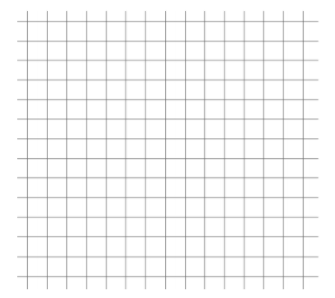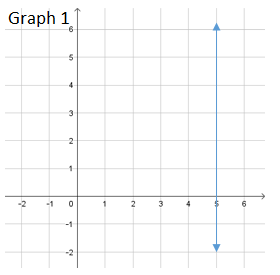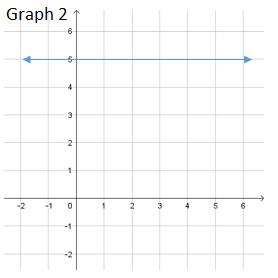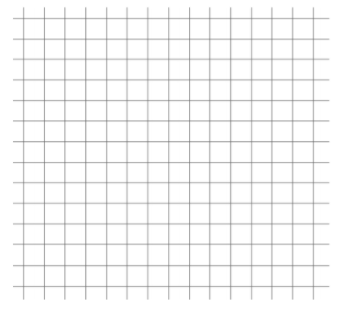# Linear Relationships

## Objective

Write equations into slope-intercept form in order to graph. Graph vertical and horizontal lines.

## Common Core Standards

### Core Standards

?

• 8.EE.B.6 — Use similar triangles to explain why the slope m is the same between any two distinct points on a non-vertical line in the coordinate plane; derive the equation y = mx for a line through the origin and the equation y = mx + b for a line intercepting the vertical axis at b.

?

• 8.EE.C.7

## Criteria for Success

?

1. Distinguish between standard form and slope-intercept form.
2. Rewrite an equation into slope-intercept form by solving for $y$.
3. Graph a linear equation using the slope and $y$-intercept.
4. Understand that the equation $y=a$, where a is a constant, is a horizontal line with a zero slope, and the equation ${x=a}$ is a vertical line with undefined slope.

## Tips for Teachers

?

Lesson 9 is a continuation of Lesson 8 but presents equations in standard form that are then written into slope-intercept form as a strategy to graph.

### Remote Learning Guidance

If you need to adapt or shorten this lesson for remote learning, we suggest prioritizing Anchor Problems 2 and 3 (benefit from worked examples). Find more guidance on adapting our math curriculum for remote learning here.

#### Fishtank Plus

Subscribe to Fishtank Plus to unlock access to additional resources for this lesson, including:

• Problem Set
• Student Handout Editor
• Google Classrom Integration
• Vocabulary Package

## Anchor Problems

?

### Problem 1

Given the equation ${2x+3y=9}$, determine the slope and $y$-intercept, and then graph the line that represents the equation.#### Guiding Questions

Create a free account or sign in to access the Guiding Questions for this Anchor Problem.

### Problem 2

Write the equation below into slope-intercept form and then graph the line that represents the equation.

${-4x-2y=-5}$#### Guiding Questions

Create a free account or sign in to access the Guiding Questions for this Anchor Problem.

### Problem 3

Match each equation to a graph. Be prepared to explain your reasoning.

Equation A: ${ y=5}$
Equation B: ${x=5}$#### Guiding Questions

Create a free account or sign in to access the Guiding Questions for this Anchor Problem.

## Problem Set

?With Fishtank Plus, you can download a complete problem set and answer key for this lesson. Download Sample

The following resources include problems and activities aligned to the objective of the lesson that can be used to create your own problem set.

## Target Task

?

Identify the slope and $y$-intercept of the equation $12x-8y=24$.
Then graph the line that represents the equation.Create a free account or sign in to view Mastery Response